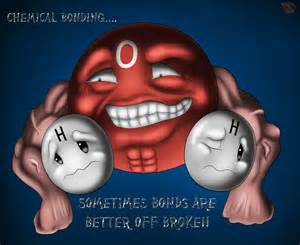Covalent Compounds
3 years ago
aslonaker
Save
Edit
Host a game
Live GameLive
Homework
Solo Practice
Practice15 QuestionsShow answers
• Question 1
30 seconds
Q. What is the prefix for 1?
mono
di
tri
tetra
• Question 2
30 seconds
Q. Name CCl4
carbon carbon tetraiodide
carbon tetrachloride
monocarbon tetrachloride
water
• Question 3
30 seconds
Q. Name BCl3
boron chloride
boron (III) chloride
boron trichloride
boron chlorine
• Question 4
30 seconds
Q. Give the formula for oxygen dichloride
OCl2

O2Cl2

OCl
O2Cl
• Question 5
30 seconds
Q. Give the formula for phosphorus trifluoride
P3F
PF
PF3
P3F3
• Question 6
30 seconds
Q. Give the formula for ammonia
NH3
NH4
CH4
H2O
• Question 7
30 seconds
Q. Name CO2
carbon dioxide
carbon monoxide
carbon oxide
monocarbon dioxide
• Question 8
30 seconds
Q. What is the prefix for 8?
non
tri
di
oct
• Question 9
30 seconds
Q. What is the prefix for 10?
mono
tetra
dec

hept

• Question 10
30 seconds
Q. Name SO2
monosulfur dioxide
sulfur dioxide
sulfur oxide

sulfur (II) oxide

• Question 11
30 seconds
Q. How many valence electrons does carbon have?
1
2
3

4

• Question 12
30 seconds
Q. How many valence electrons does oxygen have?
2
3
6
4
• Question 13
30 seconds
Q. How many valence electrons does chlorine have?
1
7
4
2
• Question 14
30 seconds
Q. How many valence electrons does nitrogen have?
3

2

5
1
• Question 15
30 seconds
Q. How many valence electrons does hydrogen have?